# Experiment 2.0.1

Searching for an image classification fail case by varying illumination (2nd attempt).

Carrying on from experiment 2.0.0.

## 1. Experiment improvements

This time, I make sure each input image contains an object that corresponds to an ImageNet class. Many of the images in 2.0.0 contained no objects with corresponding ImageNet labels.

I also noticed that the images have an embedded colorspace, “Linear Rec2020 RGB”, and so the images need to be converted in order to be in the colorspace expected by ResNet (sRGB). This was not done in 2.0.0, but is done here in 2.0.1.

import tempfile
import zipfile
import urllib
import numpy as np
import torch
import pathlib
import torchvision as tv
import torchvision.datasets
import torchvision.models
import torchvision.transforms
import pandas as pd
from icecream import ic
import json
import xarray as xr
import matplotlib as mpl
import matplotlib.pyplot as plt
from collections import namedtuple
import ipyplot
import cv2
import einops
import PIL
import PIL.ImageCms
import IPython

with open('./resources/imagenet-simple-labels.json') as f:
labels_to_id = {s:i for (i,s) in enumerate(labels)}

NUM_CLASSES = 1000
assert NUM_CLASSES == len(labels)

def class_id_to_label(cid):
assert int(cid) == cid
cid = int(cid)
return labels[cid]

def label_to_class_id(label):
return labels_to_id[label]

def imshow(img):
"""Show image.

Image is a HWC numpy array with values in the range 0-1."""
img = img*255
img = cv2.cvtColor(img, cv2.COLOR_RGB2BGR)
# cv2 imencode takes images in HWC dimension order.
_,ret = cv2.imencode('.jpg', img)
i = IPython.display.Image(data=ret)
IPython.display.display(i)

def imlist(images, labels=None, use_tabs=False):
if use_tabs:
ipyplot.plot_class_tabs(images, labels, max_imgs_per_tab=300)
else:
ipyplot.plot_images(images, labels)

# Choose CPU or GPU.
device = torch.device('cuda:0')
#device = "cpu"

# Choose small or large (standard) model variant
#model_name = "resnet18"
model_name = 'resnet50'
def model_fctn():
if model_name == 'resnet18':
return tv.models.resnet18(pretrained=True)
elif model_name == 'resnet50':
return tv.models.resnet50(pretrained=True)
model = model_fctn()
model = model.to(device)
model.eval()

def model_name_str():
"""Returns the printable string form of the model."""
res = None
if model_name == 'resnet18':
res = 'ResNet-18'
elif model_name == 'resnet50':
res = 'ResNet-50'
else:
raise Exception('Unexpected model.')
return res

IMG_SHAPE = (224, 224, 3)

Downloading: "https://download.pytorch.org/models/resnet50-19c8e357.pth" to /home/app/.cache/torch/hub/checkpoints/resnet50-19c8e357.pth

HBox(children=(HTML(value=''), FloatProgress(value=0.0, max=102502400.0), HTML(value='')))

ds_path = pathlib.Path('resources/exp_2/mls_dataset')
def is_empty(path):
return not any(path.iterdir())
ds_path.mkdir(exist_ok=True)
zip_path, _ = urllib.request.urlretrieve('ftp://vis.iitp.ru/mls-dataset/images_preview.zip')
with zipfile.ZipFile(zip_path, "r") as f:
f.extractall(ds_path)


## 2. Dataset

The dataset is constructed by extracting crops from the following 24 scenes, repeated in 18 different illuminations. Dataset is the mls-dataset: https://github.com/Visillect/mls-dataset.

The crops are hand chosen to insure that each image can be meaningfully labeled with 1 of the 1000 ImageNet labels. The cropped images are transformed again as a form of data augmentation. The data augmentation carries out a 5-crop transform, outputting 5 cropped images for each input image.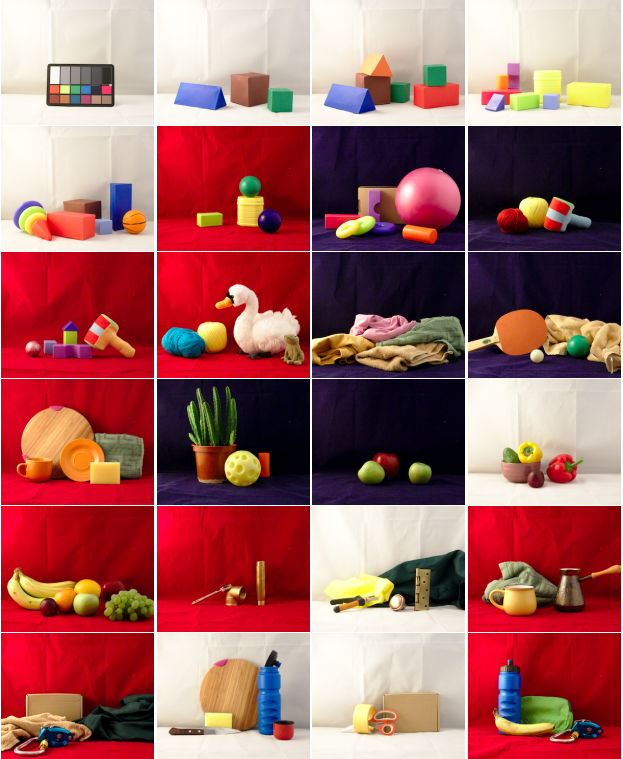The next code section prepares the dataset. The dataset images are printed at the end.

# Transforms
normalize_transform =  tv.transforms.Normalize([0.485, 0.456, 0.406], [0.229, 0.224, 0.225])
crop = tv.transforms.FiveCrop(size=IMG_SHAPE)
pre_norm_transform = tv.transforms.Compose([crop])
norm_transform = tv.transforms.Compose([normalize_transform])

# Data details
num_scenes = 24
ignore_scenes = {1,} # 0: the Macbeth chart
scenes = [s for s in range(1, num_scenes+1) if s not in ignore_scenes]
crops = ['topleft', 'topright', 'bottomleft', 'bottomright', 'center']
Crop = namedtuple('Crop', 'y, x, h, w')
Scene = namedtuple('Scene', 'imagenet_label, id, crop')
# Choose a crop size that allows for 5 sub-crops of size 224.
crop_size = int(224 * 3/2) # 336
scenes = [
Scene('bath towel',      11, Crop(157, 264, crop_size, crop_size)),
Scene('ping-pong ball',  12, Crop(230, 110, 263,       292)),       # Image made smaller to keep ball in all crops.
Scene('cup',             13, Crop(157,   0, crop_size, crop_size)), # Not sure if 'cup' or 'coffee mug' is the best class.
Scene('pot',             14, Crop(117,  50, crop_size, crop_size)),
Scene('Granny Smith',    15, Crop(185, 264, crop_size, crop_size)),
Scene('bell pepper',     16, Crop(157, 216, crop_size, crop_size)),
Scene('banana',          17, Crop(157,   0, crop_size, crop_size)), # This image also contains an orange and apple.
Scene('coffee mug',      20, Crop(197,  15, 296,       crop_size)), # Image made to keep the cup in all crops.
Scene('water bottle',    22, Crop( 65, 264, crop_size, crop_size)),
Scene('water bottle',    24, Crop( 65,   0, crop_size, crop_size)), # This image also contains a banana.
Scene('banana',          24, Crop(197,  50, 296,       crop_size))] # This image also contains a water bottle.
illuminants = [
'2HAL_DESK_LED-B025',
'2HAL_DESK_LED-B050',
'2HAL_DESK_LED-B075',
'2HAL_DESK_LED-B100',
'2HAL_DESK_LED-BG025',
'2HAL_DESK_LED-BG050',
'2HAL_DESK_LED-BG075',
'2HAL_DESK_LED-BG100',
'2HAL_DESK_R025',
'2HAL_DESK_R050',
'2HAL_DESK_R075',
'2HAL_DESK_R100',
'2HAL_DESK_RG025',
'2HAL_DESK_RG050',
'2HAL_DESK_RG075',
'2HAL_DESK_RG100',
'2HAL_DESK',
'2HAL']

def img_key(scene_id, imagenet_label, crop_label, illuminant):
return f'{scene_id}.{imagenet_label}.{crop_label}.{illuminant}'

def rec_2020_to_sRGB(rgb_img):
"""Convert image in REC2020 linear colorspace to an sRGB colorspace image.

This method didn't actually seem to work, yet I'm not exactly sure why, so
I'm keeping it here so I can come back and try understand why it isn't working.
Below, I ended up using the inbuilt features of PIL instead.
"""
# Rec 2020 to CIE XYZ
to_xyz_mat = torch.tensor([[6.36953507e-01, 1.44619185e-01, 1.68855854e-01],
[2.62698339e-01, 6.78008766e-01, 5.92928953e-02],
[4.99407097e-17, 2.80731358e-02, 1.06082723e+00]])
def dot_vector(m, v):
return  torch.einsum('...ij,...j->...i', m, v)
xyz = dot_vector(to_xyz_mat, rgb_img)

# CIE XYZ to sRGB
to_linear_rgb_mat = torch.tensor([
[3.2406, -1.5372, -0.4986],
[-0.9689, 1.8758, 0.0415],
[0.0557, 0.2040, 1.057]])
linear_rgb = dot_vector(to_linear_rgb_mat, xyz)

def to_srgb(c):
res = 12.92*c if c <= 0.0031308 else (1.055 * c**(1/2.4) - 0.055)
return res
s_rgb = linear_rgb.apply_(to_srgb)
s_rgb_chw = einops.rearrange(s_rgb, 'h w c -> c h w')
return s_rgb_chw

def open_as_srgb(img_path):
"""Open an image and convert it to sRGB.

The image must have an embedded ICC color profile."""
img = PIL.Image.open(img_path)
icc = tempfile.mkstemp(suffix='.icc')
with open(icc, 'wb') as f:
f.write(img.info.get('icc_profile'))
srgb = PIL.ImageCms.createProfile('sRGB')
img = PIL.ImageCms.profileToProfile(img, icc, srgb)
return img

def open_img(scene, illuminant):
"""Open the image corresponding to the given scene and illuminant."""
img_path = ds_path / 'images_preview' / f'{scene.id:02d}' / f'{scene.id:02d}_{illuminant}.jpg'
img = open_as_srgb(img_path)
img = np.array(img, dtype=np.float32)
cropped = img[scene.crop.y:scene.crop.y+scene.crop.h, scene.crop.x:scene.crop.x+scene.crop.w, :]
return cropped

def create_dataset():
"""
Dataset as a dict. Keys are of the form: 04-topleft-2HAL_DESK_LED-B025.
Images are 0-1 tensors.
"""
images = dict()
for s in scenes:
for ill in illuminants:
img = open_img(s, ill)
#img = np.asarray(img, dtype=np.float32) / 255.0
img = img / 255.0
img = torch.tensor(einops.rearrange(img, 'h w c -> c h w'))
cropped_images = pre_norm_transform(img)
for crop_label, ci in zip(crops, cropped_images):
images[img_key(s.id, s.imagenet_label, crop_label, ill)] = ci
return images

ds = create_dataset()

def get_ds_image(ds, scene, illuminant, subcrop):
# img is torch in CxHxW format.
img = ds[img_key(scene.id, scene.imagenet_label, subcrop, illuminant)]
img = einops.rearrange(img, 'c h w -> h w c').numpy()
return img

def print_originals():
"""Print the images before the 5-crop transformation.

Only images for one illumination are printed."""
images = []
labels = []
for s in scenes:
img = open_img(s, illuminants[-2])
labels.append(s.imagenet_label)
images.append(img)
imlist(images, labels)

def print_dataset(inc_illuminants=None):
"""Print the dataset images.

Args:
inc_illuminants (set): restrict the illuminants to this set. Without
setting this option, the number of images printed
with be quite large (11x5x18).
"""
if not inc_illuminants:
inc_illuminants = set(illuminants)
ds = create_dataset()
tab_labels = []
images = []
custom_labels = []
for k,v in ds.items():
sid, imagenet_label, crop, illuminant = k.split('.')
if not illuminant in inc_illuminants:
continue
tab_labels.append(illuminant)
images.append(einops.rearrange(v.numpy(), 'c h w -> h w c'))
custom_labels.append(f'{imagenet_label} ({crop})')

ipyplot.plot_class_tabs(images, tab_labels, custom_labels, max_imgs_per_tab=200)


The following images are the hand-chosen 11 different 336x366 crops, under 2HAL illumination (two halogen lights).

One aspect of the dataset that is worth pointing out is that they use a naming convention for their illuminants, so 2HAL means 2 halogen lights, DESK means a tungsten desk lamp, LED-B025 means a blue LED light at 25% power. Check the dataset source for more details.

print_originals()

/opt/conda/lib/python3.8/site-packages/numpy/core/_asarray.py:83: VisibleDeprecationWarning: Creating an ndarray from ragged nested sequences (which is a list-or-tuple of lists-or-tuples-or ndarrays with different lengths or shapes) is deprecated. If you meant to do this, you must specify 'dtype=object' when creating the ndarray
return array(a, dtype, copy=False, order=order)


#### bath towel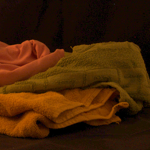#### ping-pong ball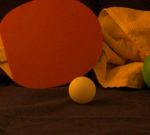#### cup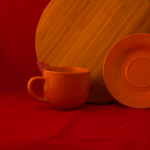#### pot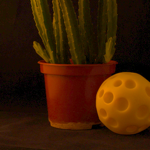#### Granny Smith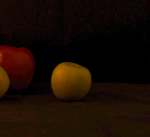#### bell pepper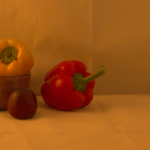#### banana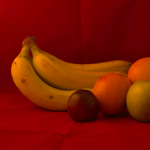#### coffee mug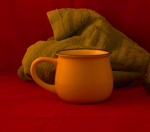#### water bottle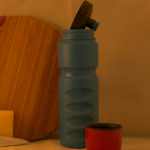#### water bottle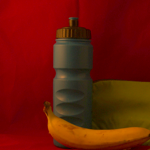#### banana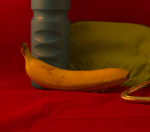Below, is a subset of the whole dataset. The 11 336x336 are cropped into 5 different 224x224 images, and this is done for all 18 illuminations. Below, only 3 out of the 18 illuminations are shown.

inc_illuminants={'2HAL_DESK', '2HAL_DESK_RG075', '2HAL_DESK_LED-B100'}
print_dataset(inc_illuminants)